# understanding operators in dynamo

image by cowgirl

Just today(yesterday?) I was working on some awesome Dynamo stuff and ran into a wall – however briefly – but I was stomped by Dynamo’s operators and their logic. I decided to explain what the operators are and how they work in Dynamo as well as how they work in Code Blocks because they have the most power there. Below is a list of operators available to us in Dynamo, some of them basic and pretty straight forward and some a little more complicated.

The most basic operator is an Assignment Operator (=). You can use it to assign a value to a variable: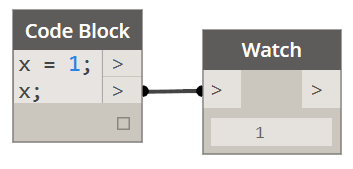You can also use it to assign one variable to another variable:We can get a little funky with the assignment operators and assign values to variables while performing some other operation on another variable:Now, for some reason the following is not possible in Dynamo’s Code Block. I shall ask the Dynamo team why at some later time, but theoretically you should also be able to do this:Finally you can also assign same value to multiple variables using the following syntax:The next set of operators available in Dynamo is probably the most recognizable one: The Arithmetic Operators (+, -, *, /, %). These are the same operators that a standard C++ supports. They are pretty straight forward so I am not going to explain what 2 + 2 evaluates to. :-).

• +  |  addition
• =  | subtraction
• * | multiplication
• / | division
• % | modulo – returns a reminder of a division of two values. Please see below: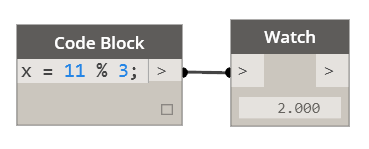Another set of Operators are Compound Assignment (+=, -=, *=, /=, %=, >>=, <<=, &=, ^=, |=). Now those are looking a lot more interesting so let’s break them down a little more. Compound operators are relatively easy to understand. They are equivalent of assigning the result of an operation to the first operand:

• x += y is the same as x = x + y
• x -=5 is the same as x = x – 5
• x *= y + 1 is the same as x = x * (y + 1)

Let’s look an example in Dynamo…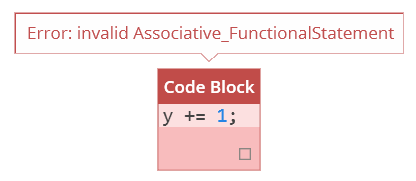…and what a party pooper that thing is, it doesn’t work yet again. As it happens none of the Compound Operators work in Code Blocks, yet/or they have some unknown to me syntax which would not surprise me.

Let’s move on and see if we can use Increment and Decrement Operators (++, –).Ayayaya…the errors keep piling up, and I am starting to wonder what is with you Design Script? Anyways, let’s move on and let’s hope that someone from the Dynamo team will read this one day and explain to me how all these operators are supposed to function in Design Script.

Relational and Comparison Operators are next (==, !=, >, <, >=, <=). Two values can theoretically (that remains to be seen) be compared to see if they are equal using the following syntax: x == y. Here’s the rest of them:

• == | Equal to
• != | Not Equal to
• < | Less than
• > | Greater than
• <= | Less than or equal to
• >= | Greater than or equal toNice! We are back in business. These are straight up numerical comparisons, but let’s do something with variables:As you can see since x is equal to 3 it returns false when compared to 5. It’s all working well. One thing to keep in mind is that a single = operator is not the same as double ==. As you noticed one assigns a value while the other compares to arguments on each side of it.

Our next set of operators are the Logical Operators (!, &&, ||). Now the ! operator is the same as saying NOT while && are equivalent of AND and finally || are the OR operator. These are extremely important and quite useful operators. Let’s have a closer look at them.Why do you think this statement reads False? Well, let’s step through it step by step from right to left: First we do a comparison asking if 5 is equal to 5. This will evaluate to True but then we say NOT which reverses the True statement and returns our final result which is a False.

Let’s look at one more example: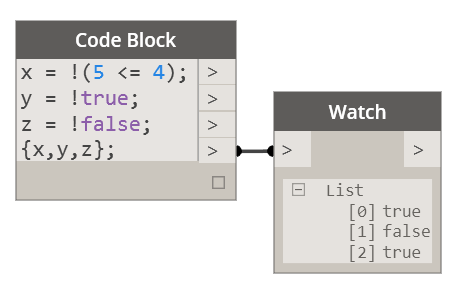Of course 5 is not less than 4 so reverse of that is True. Same with the next one where reverse of True is False. Finally reverse of False is True.

Let’s have a look at the AND operator:In case of the AND operator both of the variables have to be True for it to return a True. If any of the operands is False then && operation will return False. Please see below: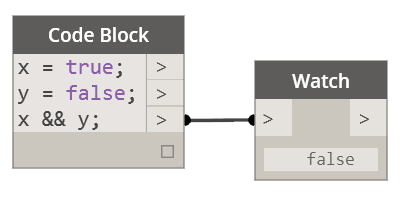Now let’s look at the OR operator which would have behave a little bit differently in this case: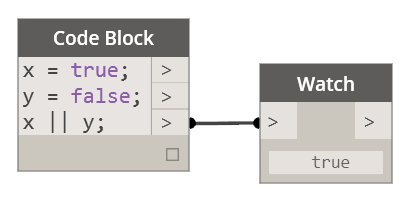As you can see the OR operator will return True if ANY of the operands is True. It will only return false if BOTH of the operands are False: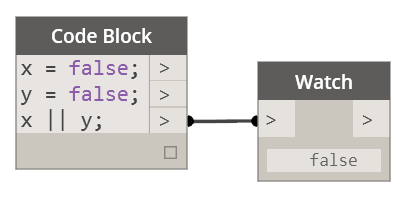This next operator is quite interesting. It’s called Ternary Operator (?). What it does is that it evaluates an expression and depending on whether it evaluates to True it returns one value or a different one for false: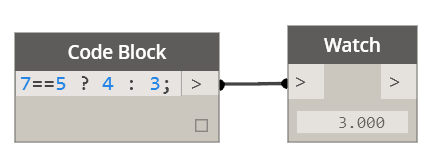You can see how the 7 equals to 5 returns False so the final value returned is 3. The way this operator works is the following:

• condition ? result1 (if True) : result2 (if False)

In this case the condition was false so we got result2 back. Pretty slick operator that combines a simple comparison operator and an IF statement. What if we used variables with it?This is pretty slick. We compare a length of one list to another and return something that is totally unrelated to either.

Our next operator is a Comma Operator (,). The comma operator is used to separate two or more expressions that are included where only one expression is expected. For example: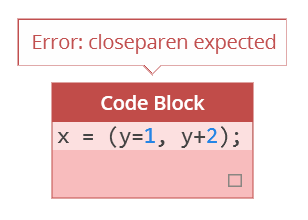Oh well, someone please help. I am dying here with this Design Script syntax issues. I need to learn me some DS.

Well I thought this would be useful, but it seems like half of them didn’t work either because of my lack of Design Script competency or because they are not yet implemented in Dynamo. Either way, I will be happy to get schooled on proper use of all of the above operators. #Qs4Colin?

All operator logic based on original post here: cplusplus